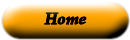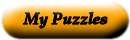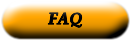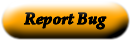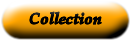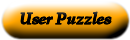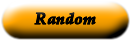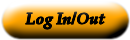apply a set of rules for writing code that a computer can evaluate arguments information from the computer about errors in code circle of evaluation software in which you can write and evaluate code editor the result of computing an expression error message code that follows the Code Rules for a language evaluate he inputs to a function; expressions for arguments follow the name of a function evaluates to a computation written in the rules of some language (such as arithmetic, code, or a Circle of Evaluation) expression a diagram of the structure of an expression (arithmetic or code) function a specific piece of data, like 5 or "hello" legal expression a mathematical object that takes in some inputs and produces an output mathematical expression perform the computation in an expression, producing an answer programming language use a given function on some inputs value: a computation in arithmetic

Use the "Printable HTML" button to get a clean page, in either HTML or PDF, that you can use your browser's print button to print. This page won't have buttons or ads, just your puzzle. The PDF format allows the web site to know how large a printer page is, and the fonts are scaled to fill the page. The PDF takes awhile to generate. Don't panic!Web armoredpenguin.com